# Designed Working Period of a Hoist

Date: 10/01/2019

## DESIGNED WORKING PERIOD (DWP) CALCULATION

The end of the Designed Working Period (DWP) must be calculated in accordance with the ISO 12482-1 standard during each recurring inspection and service. If the component does not have a condition monitoring unit, use the following method to calculate the remaining DWP%.

Note: If a condition monitoring unit is fitted, it performs the DWP calculation and displays the remaining DWP% automatically.

Note: For some products, the condition monitoring unit can be retrofitted as a modernization to enhance safety (not available for chain hoists). Contact your supplier for more details.

Note: The values used in each DWP calculation, as well as the result and date, must be carefully recorded in the log book (provided with the equipment). Each DWP calculation requires the use of figures recorded during the previous calculations.

Step 1: Calculate the motor operating hours (running hours) per inspection interval, T

Check the following values for this inspection interval:

J = the number of working days during the inspection interval [days]

H = the average hoisting height [m]

N = the average number of work cycles per hour [cycles/h]

T = the average daily working time [h]

V = the maximum hoisting speed [m/min] (as shown on the date plate)

Use the follwing formula to calculate Ti, the motor operating hours (total lifting time) per inspection interval: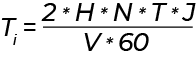For example, if we use:

J = 180 [days], H = 5 [m], = 20 [cycles/h], T = 12 [h], V = 5 [m/min]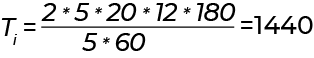Step 2: Calculate the actual load spectrum factor per inspection interval, Kmi

1. Divide the total lifting time proportion to the actual load spectrum during the inspection interval. For example, if the product has lifted full loads (100%) for half of the time and no load (0%) for half of the time then record 50 for each of these in the "Lifting time %" column of the table below.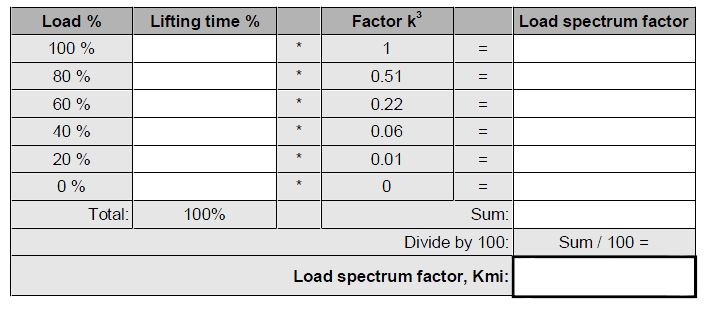2. The sum of the figures in the "Lifting time %" column must always be 100.

3. Multiply each entry in the "Lifting time %" colum by the multiplier in the "Factor k3" column. Write the results in the "Load spectrum factor" column.

4. Add up the numbers in the "Load spectrum factor" column and record the result of this sum.

5. Divide the sum of the "Load spectrum factor" column by 100 to get the Kmi

For example, if we use: 100% load for 10% of the time, 40% load for 30% of the time and 20% load for 60% of the time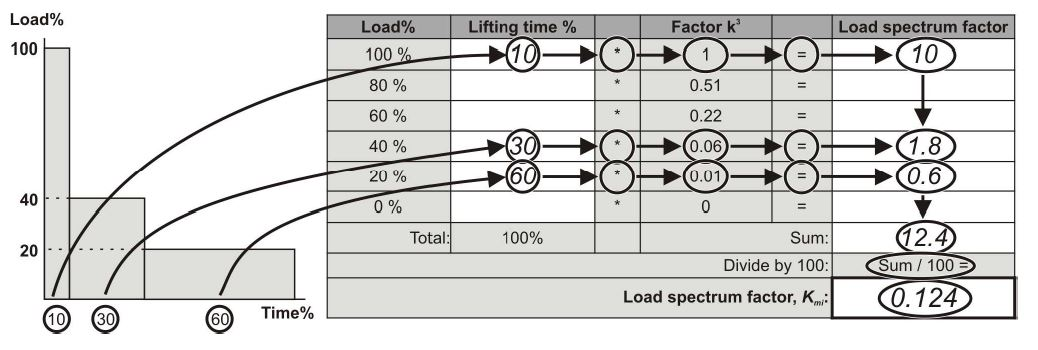Step 3: Calculate the partial duration of service, Si

Use Ti and Kmi in the following formula to calculate Si [hours]

Select the value of X, from the table below.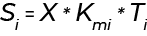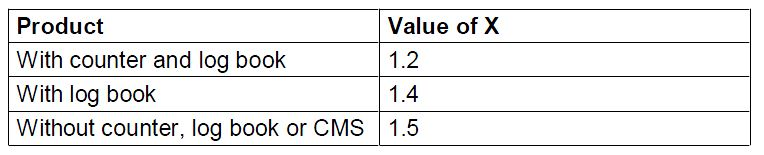Record the value of Si in the log book. This value will be needed for future DWP calculations.

For example: if we use: X = 1.2, Kmi = 0.124 and Ti = 1440: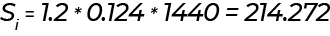Step 4: Calculate the actual duration of service, S

Add together each of the Si partial duration of service values gathered from this and previous inspection intervals since the start of the Designed Working Period.

The earlier S values (S1...Si) can be read from the log book.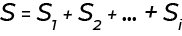For example, if we use S1 = 215.468 , S2 = 210.26, S3 (Si) = 214.272: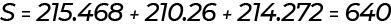Step 5: Calculate the DWP% and remaining service life.

Check the hoist operating group which can be found on the hoist's rating plate.

In the appropriate column of the following table, find the number closest to S. The two final columns on the same row will tell you the remaining DWP% and the estimated remaining service life.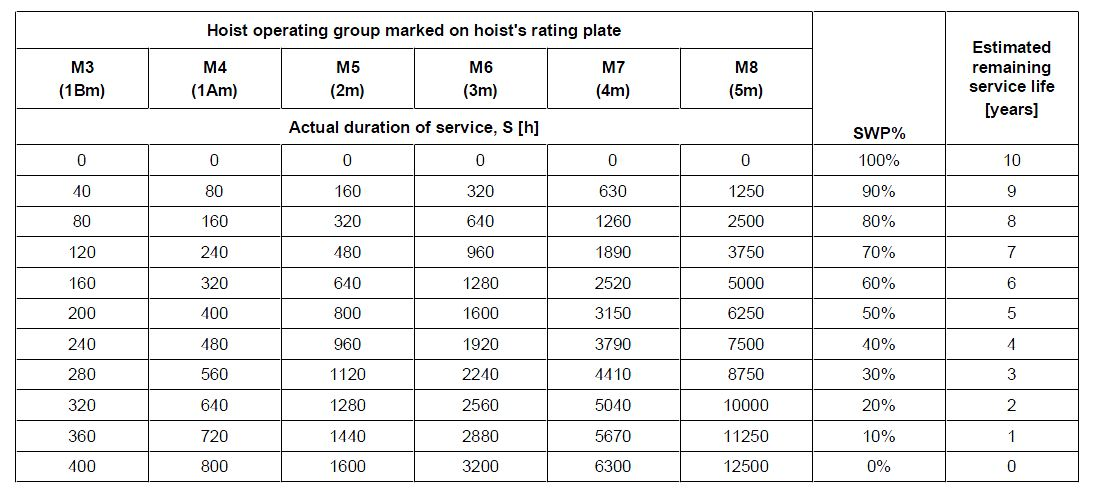Record the value of DWP% in the log book.

For example, if we use S = 640. Hoist duty group = M5 (2m) then DWP% = 60%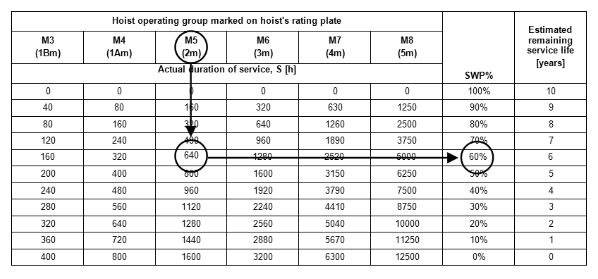When DWP% reaches zero, a General Overhaul (GO) must be conducted. Refer to chapter "General Overhaul (GO)" in your owner's manual.